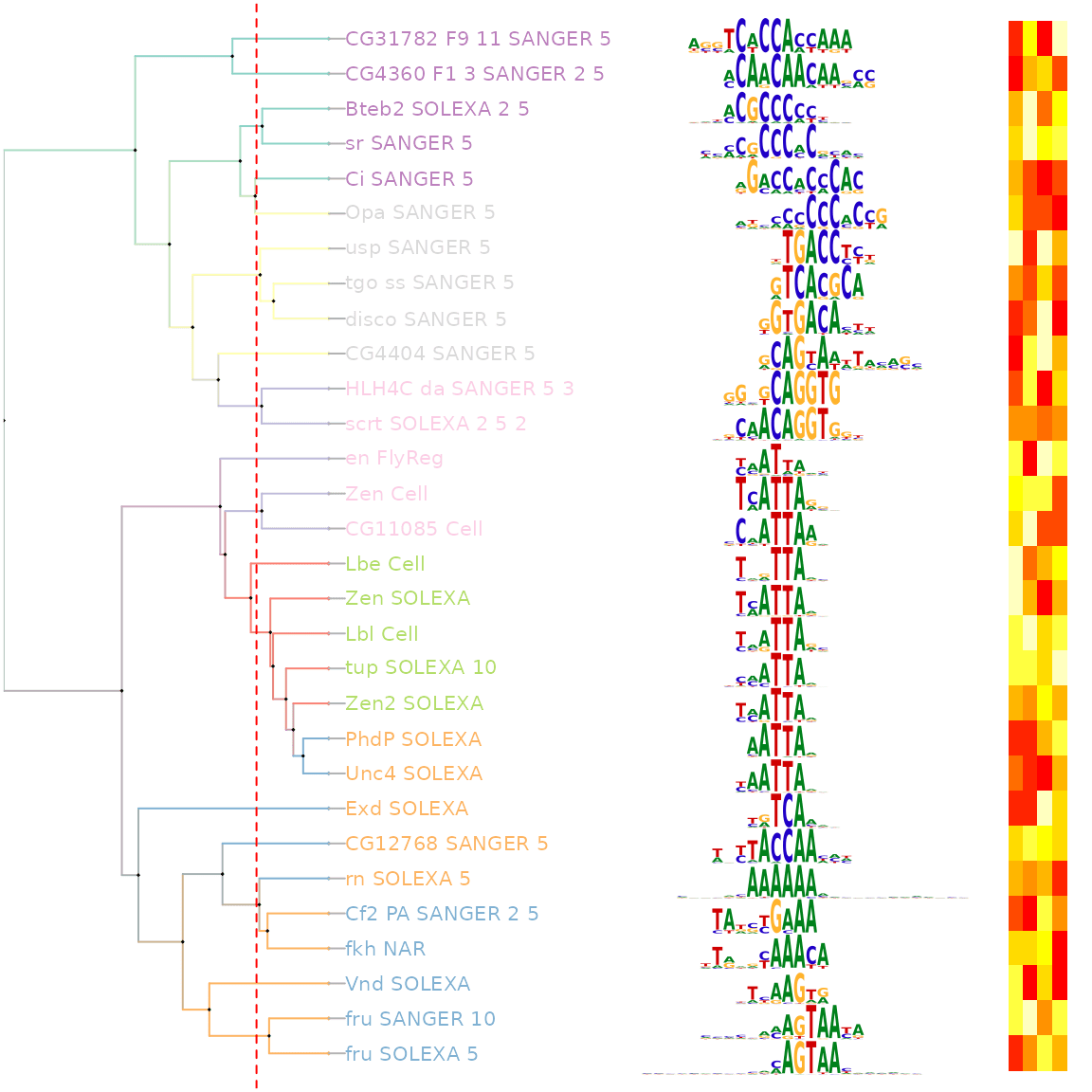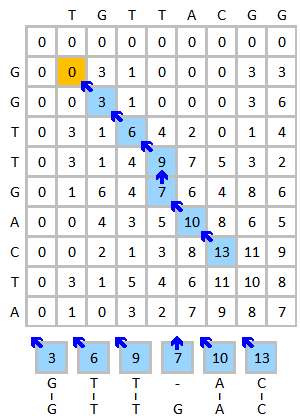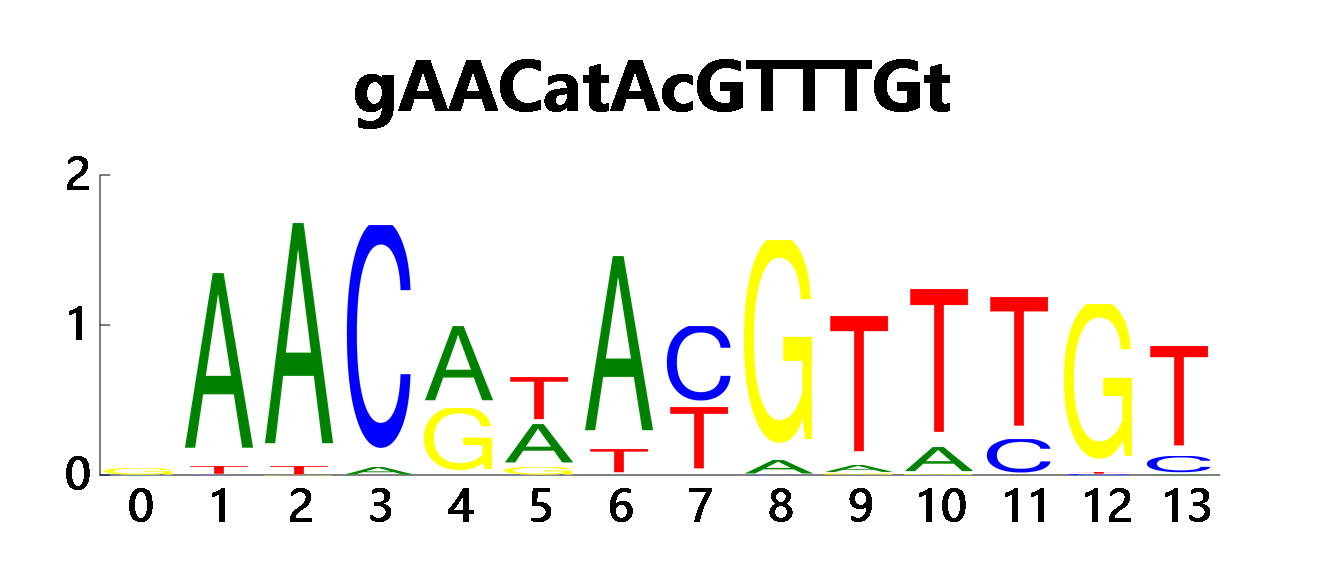Motif是一段典型的序列或者一个结构。一般情况下是指构成任何一种特征序列的基本结构。通俗来讲，即是有特征的短序列，一般认为它是拥有生物学功能的保守序列，可能包含特异性的结合位点，或者是涉及某一个特定生物学过程的有共性的序列区段。比如蛋白质的序列特异性结合位点，如核酸酶和转录因子。

## Motif位点的无监督聚类

### 局部最优比对种子

Dim seeds As List(Of HSP) = regions _
.AsParallel _
.Select(Function(q) regions.seeding(q, param)) _
.IteratesALL _
.AsList

Public Function pairwiseSeeding(q As FastaSeq, s As FastaSeq, param As PopulatorParameter) As IEnumerable(Of HSP)
Dim smithWaterman As New SmithWaterman(q.SequenceData, s.SequenceData, New DNAMatrix)
Call smithWaterman.BuildMatrix()
Dim result = smithWaterman.GetOutput(param.seedingCutoff, param.minW)
Return result.HSP.Where(Function(seed) seed.LengthHit <= param.maxW)
End Function

pairwiseSeeding函数中，我们对任意两条序列进行局部最优比较，可以得到一系列的高分区片段。对得到的高分区候选，按照一定的长度做一下过滤之后，就可以用于下游的聚类分析了。Ou, J., Wolfe, S., Brodsky, M. et al. motifStack for the analysis of transcription factor binding site evolution. Nat Methods 15, 8–9 (2018). https://doi.org/10.1038/nmeth.4555

### 二叉树聚类

Dim tree = seeds _
.Select(Function(q) New NamedValue(Of String)(q.Query, q.Query)) _
.BuildAVLTreeCluster(param.seedingCutoff)

<Extension>
Public Function BuildAVLTreeCluster(seeds As IEnumerable(Of NamedValue(Of String)), Optional cutoff# = 0.95) As BinaryTree(Of String, String)
Dim divid# = cutoff / 2
Dim cluster As New AVLTree(Of String, String)(
Function(q, s)
Dim SSM# = SeedCluster.Compare(q, s)

If SSM >= cutoff Then
Return 0
ElseIf SSM >= divid Then
Return 1
Else
Return -1
End If
End Function, Function(s) s)

For Each seed As NamedValue(Of String) In seeds
Next

Return cluster.root
End Function

<Extension>
Public Function ScoreVector(compares As (q$, s$)) As (q As Vector, s As Vector)
Dim query As New FastaSeq With {.SequenceData = compares.q.ToUpper, .Headers = {"query"}}
Dim subject As New FastaSeq With {.SequenceData = compares.s.ToUpper, .Headers = {"subject"}}
Dim globalAlign As GlobalAlign(Of Char) = RunNeedlemanWunsch.RunAlign(query, subject, 0).First
Dim q = globalAlign.query.AsEnumerable
Dim s = globalAlign.subject.AsEnumerable
Dim a As New List(Of Double)
Dim b As New List(Of Double)

For Each nt As SeqValue(Of (q As Char, s As Char)) In (q, s).SeqTuple
With nt.value.ScoreTuple
End With
Next

Return (a.AsVector, b.AsVector)
End Function

### 产生PWM矩阵

<Extension>
Private Function motif(group As BinaryTree(Of String, String),
regions As FastaSeq(),
param As PopulatorParameter) As SequenceMotif

Dim members As List(Of String) = group!values
Dim MSA As MSAOutput = members _
.Select(Function(seq)
Return New FastaSeq With {
.SequenceData = seq,
}
End Function) _
.MultipleAlignment(Nothing)
Dim PWM As SequenceMotif = MSA.PWM(members:=regions, param:=param)

Return PWM
End Function

Dim residues As New List(Of Residue)
Dim nt = {"A"c, "T"c, "G"c, "C"c}
Dim MSA As String() = alignment.MSA

For i As Integer = 0 To MSA(Scan0).Length - 1
Dim index% = i
Dim P = MSA _
.Select(Function(seq) seq(index)) _
.GroupBy(Function(c) c) _
.ToDictionary(Function(c) c.Key,
Function(g)
Return g.Count / MSA.Length
End Function)
Dim Pi = nt.ToDictionary(
Function(base) base,
Function(base)
Return P.TryGetValue(base)
End Function)

residues += New Residue With {
.frequency = Pi,
.index = i
}
Next

## Motif序列位点扫描方法

### Smith-Waterman局部最优比对方法

Private Shared Function SymbolProvider(blosum As Blosum) As GenericSymbol(Of Char)
Return New GenericSymbol(Of Char)(
equals:=Function(x, y) x = y,
toChar:=Function(x) x,
empty:=Function() "-"c
)
End FunctionDim symbol As New GenericSymbol(Of Residue)(
equals:=Function(a, b) Compare(a, b) >= 0.85,
)

Public Structure Residue
Public Property frequency As Dictionary(Of Char, Double)
Public Property index As Integer
End Structure

### 使用GCModeller进行Motif发现

''' <summary>
''' find possible motifs of the given sequence collection
''' </summary>
''' <param name="fasta"></param>
''' <param name="minw%"></param>
''' <param name="maxw%"></param>
''' <param name="nmotifs%"></param>
''' <param name="noccurs%"></param>
''' <returns></returns>
<ExportAPI("find_motifs")>
Public Function GetMotifs(<RRawVectorArgument> fasta As Object,
Optional minw% = 6,
Optional maxw% = 20,
Optional nmotifs% = 25,
Optional noccurs% = 6,
Optional env As Environment = Nothing) As SequenceMotif()

Dim param As New PopulatorParameter With {
.maxW = maxw,
.minW = minw,
.seedingCutoff = 0.95,
.ScanMinW = 6,
.ScanCutoff = 0.8
}
Dim motifs As SequenceMotif() = GetFastaSeq(fasta, env) _
.PopulateMotifs(
leastN:=noccurs,
param:=param
) _
.OrderByDescending(Function(m) m.score / m.seeds.MSA.Length) _
.Take(nmotifs) _
.ToArray

Return motifs
End Function# ["LexA.fasta"]
seq
|> find_motifs(minw = 6, maxw = 10)
|> lapply(function(motif) {
motif
|> json(compress = FALSE)
|> writeLines(con = ${export}/${motifString(motif)}.json)
;

bitmap(file = ${export}/${motifString(motif)}.png) {
plot(motif);
};
})
;Latest posts by 谢桂纲 (see all)

### Order by Date Name Attachments

#### One response

1. […] 对于我而言，RegPrecise数据库是一个比较好用的转录调控因子数据库。在这个数据库里面其主要记录了原核生物细胞内的调控因子和对应的转录因子结合位点的信息。基于这些信息，我们就可以对我们所搜索出来的Motif信息进行相关搜索注释。 […]

来自中国
1.2.3.查询字符串以["起始，以"]结尾： SELECT * FROM biodeepdb_full.metabolite WHERE INSTR(name, '["') = 1 AND INSTR(name, '"]') = LENGTH(name) - LENGTH('"]') + 1 ORDER…

4.删除mysql表中重复的行数据  delete t1 from metabolite t1 where t1.id in (select t2.id from (select id, name`, formula, hashcode2, row_number() over(partition…

5.